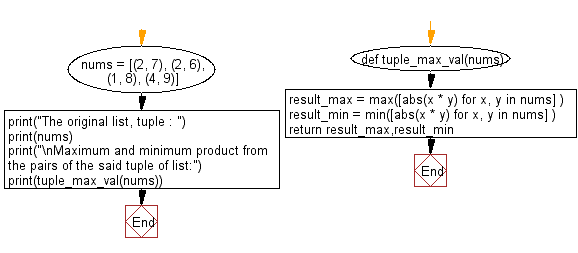﻿ Python: Find the maximum and minimum product from the pairs of tuple within a given list - w3resource# Python: Find the maximum and minimum product from the pairs of tuple within a given list

## Python List: Exercise - 124 with Solution

Write a Python program to find the maximum and minimum product from the pairs of tuple within a given list.

Sample Solution:

Python Code:

``````def tuple_max_val(nums):
result_max = max([abs(x * y) for x, y in nums] )
result_min = min([abs(x * y) for x, y in nums] )
return result_max,result_min
nums = [(2, 7), (2, 6), (1, 8), (4, 9)]
print("The original list, tuple : ")
print(nums)
print("\nMaximum and minimum product from the pairs of the said tuple of list:")
print(tuple_max_val(nums))
```
```

Sample Output:

```The original list, tuple :
[(2, 7), (2, 6), (1, 8), (4, 9)]

Maximum and minimum product from the pairs of the said tuple of list:
(36, 8)
```

Flowchart:## Visualize Python code execution:

The following tool visualize what the computer is doing step-by-step as it executes the said program:

Python Code Editor:

Have another way to solve this solution? Contribute your code (and comments) through Disqus.

What is the difficulty level of this exercise?

Test your Python skills with w3resource's quiz

﻿

## Python: Tips of the Day

Floor Division:

When we speak of division we normally mean (/) float division operator, this will give a precise result in float format with decimals.

For a rounded integer result there is (//) floor division operator in Python. Floor division will only give integer results that are round numbers.

```print(1000 // 300)
print(1000 / 300)```

Output:

```3
3.3333333333333335```